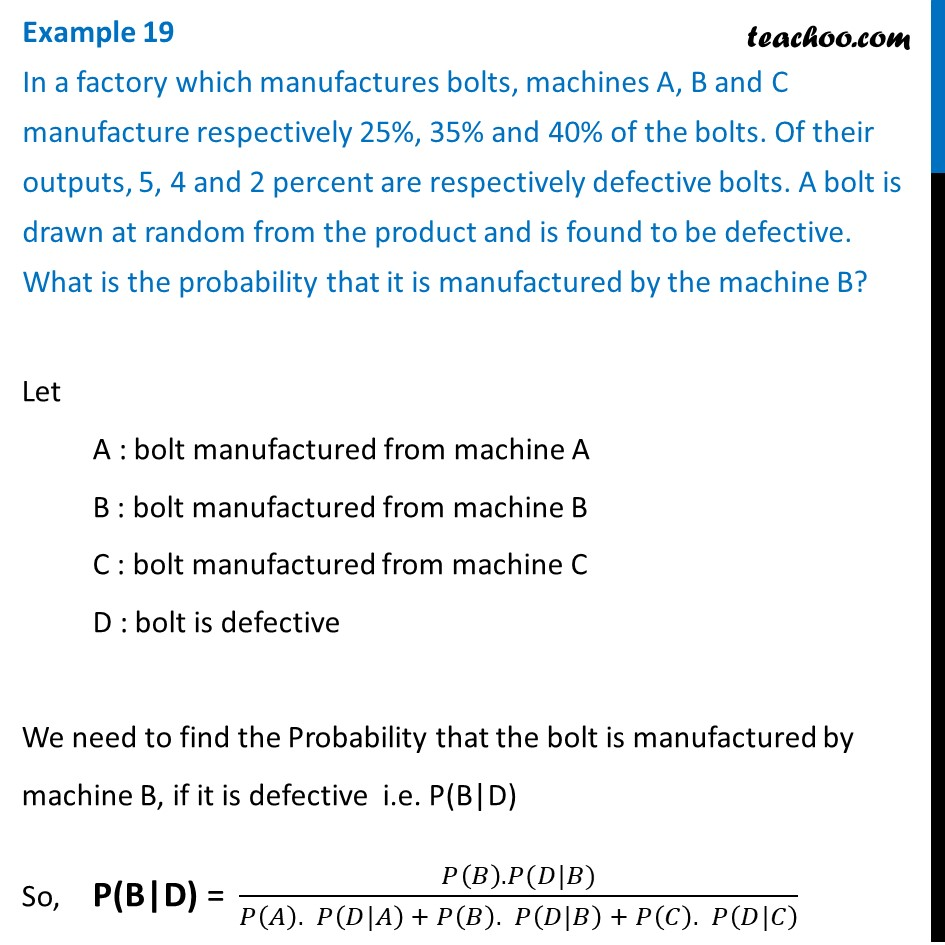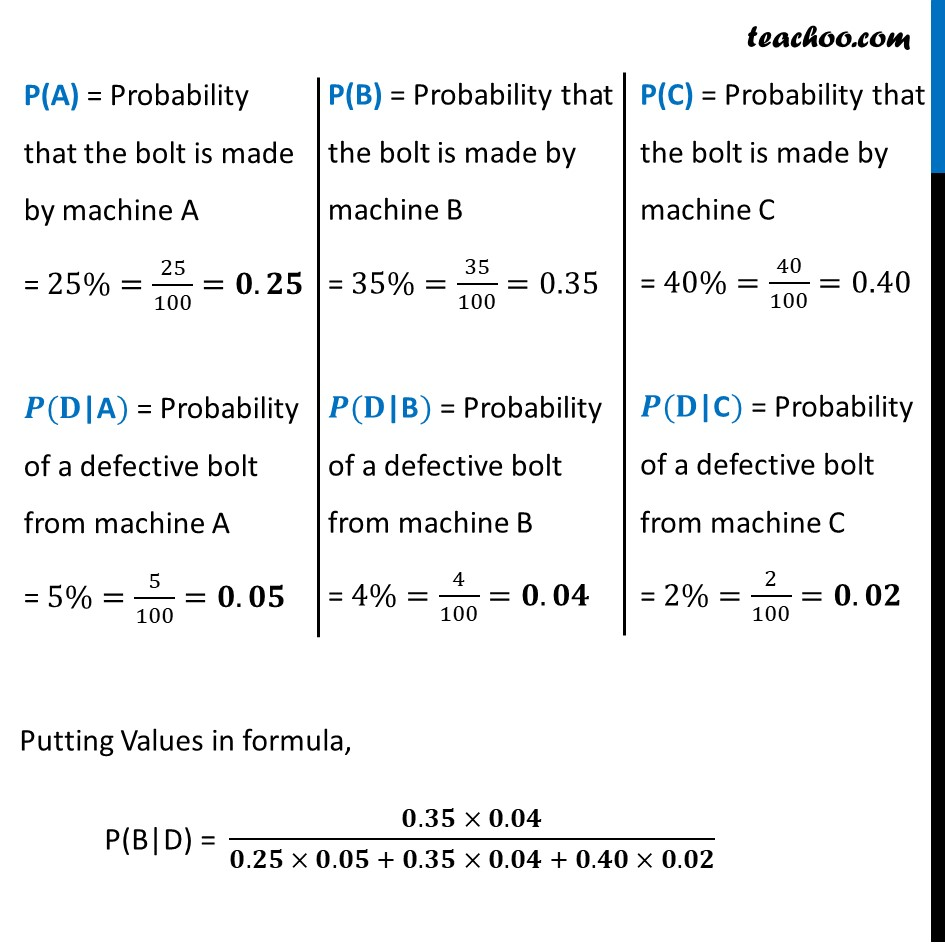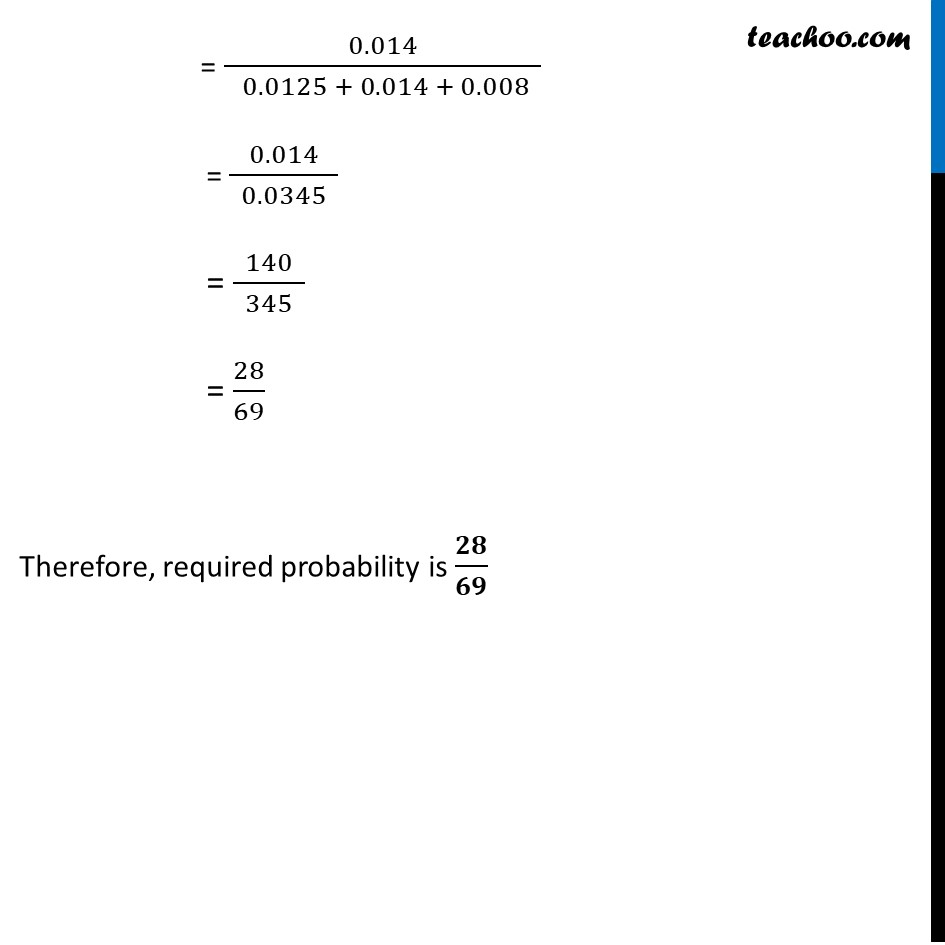Examples

Chapter 13 Class 12 Probability
Serial order wiseLearn in your speed, with individual attention - Teachoo Maths 1-on-1 Class

### Transcript

Example 19 In a factory which manufactures bolts, machines A, B and C manufacture respectively 25%, 35% and 40% of the bolts. Of their outputs, 5, 4 and 2 percent are respectively defective bolts. A bolt is drawn at random from the product and is found to be defective. What is the probability that it is manufactured by the machine B?Let A : bolt manufactured from machine A B : bolt manufactured from machine B C : bolt manufactured from machine C D : bolt is defective We need to find the Probability that the bolt is manufactured by machine B, if it is defective i.e. P(B"|"D) So, "P(B|D) = " (𝑃(𝐵). 𝑃(𝐷|𝐵))/(𝑃(𝐴). 𝑃(𝐷|𝐴) + 𝑃(𝐵). 𝑃(𝐷|𝐵) + 𝑃(𝐶). 𝑃(𝐷|𝐶) ) "P(A)" = Probability that the bolt is made by machine A = 25%=25/100=𝟎.𝟐𝟓 𝑷(𝐃"|A") = Probability of a defective bolt from machine A = 5%=5/100=𝟎.𝟎𝟓 "P(B)" = Probability that the bolt is made by machine B = 35%=35/100=0.35 𝑷(𝐃"|B") = Probability of a defective bolt from machine B = 4%=4/100=𝟎.𝟎𝟒 "P(C)" = Probability that the bolt is made by machine C = 40%=40/100=0.40 𝑷(𝐃"|C") = Probability of a defective bolt from machine C = 2%=2/100=𝟎.𝟎𝟐 Putting Values in formula, "P(B|D) = " (𝟎.𝟑𝟓 × 𝟎.𝟎𝟒)/(𝟎.𝟐𝟓 × 𝟎.𝟎𝟓 + 𝟎.𝟑𝟓 × 𝟎.𝟎𝟒 + 𝟎.𝟒𝟎 × 𝟎.𝟎𝟐) = 0.014/( 0.0125 + 0.014 + 0.008 ) = 0.014/( 0.0345 ) = 140/( 345 ) = 28/69 Therefore, required probability is 𝟐𝟖/𝟔𝟗